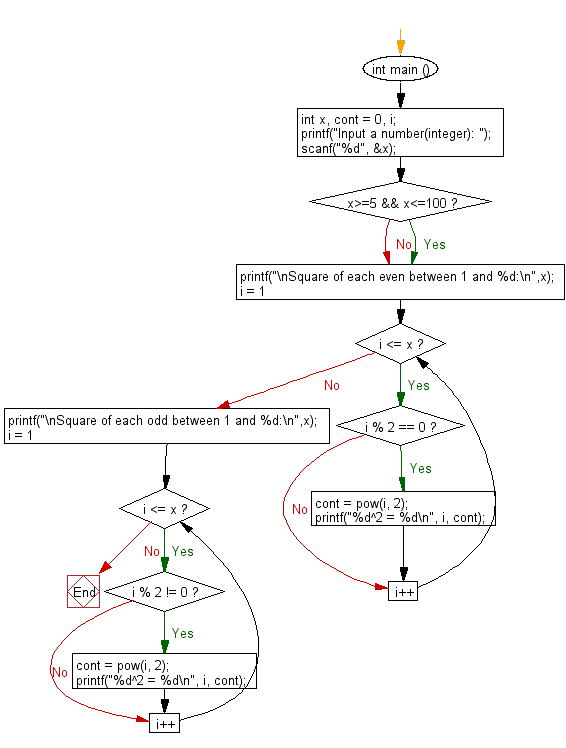﻿ C : Suare of each even and odd value between 1 and a number

# C Exercises: Find and print the square of each even and odd value between 1 and a given number

## C Basic Declarations and Expressions: Exercise-109 with Solution

Write a C program to find and print the square of each even and odd value between 1 and a given number (4 < n < 101).

Sample Solution:

C Code:

``````#include <stdio.h>
#include <math.h>

int main () {
int x, cont = 0, i;

// Prompt the user to input a number
printf("Input a number(integer): ");
scanf("%d", &x);

// Check if x is between 5 and 100
if (x >= 5 && x <= 100)
{
// Print the squares of even numbers between 1 and x
printf("\nSquare of each even between 1 and %d:\n",x);
for (i = 1; i <= x; i++){

if (i % 2 == 0){
cont = pow(i, 2);
printf("%d^2 = %d\n", i, cont);
}
}

// Print the squares of odd numbers between 1 and x
printf("\nSquare of each odd between 1 and %d:\n",x);
for (i = 1; i <= x; i++){

if (i % 2 != 0){
cont = pow(i, 2);
printf("%d^2 = %d\n", i, cont);
}
}
}
}
``````

Sample Output:

```Input a number(integer): 15

Square of each even between 1 and 15:
2^2 = 4
4^2 = 16
6^2 = 36
8^2 = 64
10^2 = 100
12^2 = 144
14^2 = 196

Square of each odd between 1 and 15:
1^2 = 1
3^2 = 9
5^2 = 25
7^2 = 49
9^2 = 81
11^2 = 121
13^2 = 169
15^2 = 225
```

Flowchart:C programming Code Editor:

What is the difficulty level of this exercise?

Test your Programming skills with w3resource's quiz.

﻿